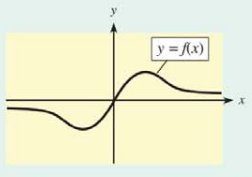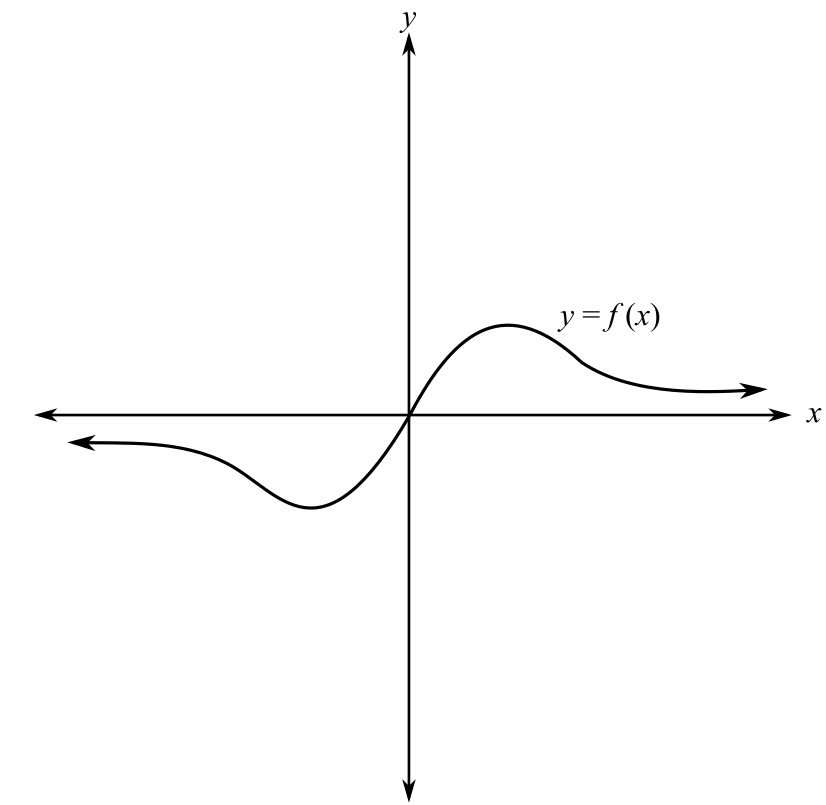Chapter 10.2, Problem 3CP### Mathematical Applications for the ...

11th Edition
Ronald J. Harshbarger + 1 other
ISBN: 9781305108042

#### Solutions

Chapter
Section### Mathematical Applications for the ...

11th Edition
Ronald J. Harshbarger + 1 other
ISBN: 9781305108042
Textbook Problem

# On the graph below, locate any points of inflection (approximately) and label where the curve satisfies f " ( x )   >   0   and  f " ( x )   < 0 .To determine

The points of inflection and the region on the curve where f(x)>0 and f(x)<0 for the provided graph of the function,Explanation

Given Information:

The provided graph of a function is,

Explanation:

Consider the provided graph,

Now, recall that the points of inflection are the values of x for which the value of the second derivative of the function is 0, that is f(x)=0.

Thus, f(x)=0 in the graph wherever the graph changes its concavity.

Mark the three points where the graph changes its concavity as A, B and C.

Since, at the points A, B and C the function satisfies f(x)=0.

Thus, the points of inflection are A, B and C.

Since, f(x) is the first derivative of f(x), wherever the graph of f(x) is increasing, it follows that f(x)>0

### Still sussing out bartleby?

Check out a sample textbook solution.

See a sample solution

#### The Solution to Your Study Problems

Bartleby provides explanations to thousands of textbook problems written by our experts, many with advanced degrees!

Get Started

#### x2 2x 5 = 0

Applied Calculus for the Managerial, Life, and Social Sciences: A Brief Approach

#### Change 885 g to mg.

Elementary Technical Mathematics

#### Evaluate the integral, if it exists. 15dt(t4)2

Single Variable Calculus: Early Transcendentals

#### Define applied research and basic research and identify examples of each.

Research Methods for the Behavioral Sciences (MindTap Course List)